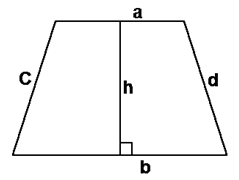# Perimeter of a Trapezoid Formula

The type of polygon or a quadrilateral having at least a pair of parallel sides is called Trapezoid. Two sides of a trapezium are parallel. The perpendicular distance between the parallel sides is called the altitude or height.The perimeter of a trapezoid formula is given by

$\large P = a + b + c + d$

Where,
a, b, c, d are the lengths of sides of a trapezoid.

### Solved Example

Question: Trapezoid ABCD has legs 4 cm and 7 cm in length. Its bases are 12 cm and 15 cm respectively. Find the perimeter of the trapezoid.

Solution:

Given:
a = 4 cm
b = 7 cm
c = 12 cm
d = 15 cm

Perimeter is given by:
P = a + b + c + d
= 4 + 7 + 12 + 15
= 38 cm